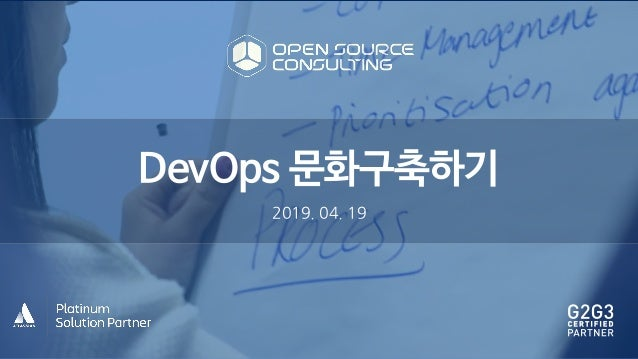Successfully reported this slideshow.Upcoming SlideShare
×

# [Atlassian meets dev ops and itsm] Building organization and collaboration culture for devops

242 views

Published on

[올바른 DevOps를 위한 조직 및 협업 문화 구축]

오픈소스컨설팅이 개최한 Atlassian meets DevOps and ITSM 세미나 발표자료를 공유합니다. 올바른 DevOps 수행을 위한 조직 및 협업 문화 구축 방법, DevOps Simulation 에 대해 확인해보실 수 있습니다.

Published in: Software
• Full Name
Comment goes here.

Are you sure you want to Yes No• Be the first to comment

### [Atlassian meets dev ops and itsm] Building organization and collaboration culture for devops

1. 1. 2H =RT r y
2. 2. 2H =RT 23C=
3. 3. 2H =RT" 23C=
4. 4. 2H =RT 2H HN ROHPU b =RHSDUL PT
5. 5. 2H =RT 2H =RT b
6. 6. 2H =RT v /JLNH o NHDP 7A n 7A   " 2H =RT o a m o b b " 2H =RT r p t m y r "
7. 7. 2H =RT /JLNH o NHDP " 7A n 7A " o b g " r f "
8. 8. 2H =RT b o 7A m o … " 2H =RT b w k r f… " m m g m l p " 2H =RT m b o 7A /UNDTTLDP   0SDG LUU /PJHNLPD 8 NLH … " t m   2H =RT k " 2H =RT … " 2H =RT m z a b im … " 2H =RT b m g y o mc n
9. 9. FVT P 1VTU OHS k m O w s d oX o wk e l n 1 NNDE SDUL P c T 2 . u ’ LPJ DTU m R m p ’ '
10. 10. 2H =RT . 23C=
11. 11.
12. 12. i a t t t a r vts C @ g k k
13. 13. . 23C=
14. 14. 2H =RT "
15. 15. r m "
16. 16. m m "
17. 17. l g p m l m n " 3 3 3 3 3 3 3 3 3 333
18. 18. 2H =RT ' m ) %) g r " B 3A :/0 %) 23C= m
19. 19. g 2H =RT r . 23C=
20. 20. 1/: SDOH SM 1VNUVSH /VU ODUL P h :HDP HDTVSH KDSH I
21. 21. DTU DLN :HDP 1KD T PMH
22. 22. 17 , 1 PULPV VT 7PUHJSDUL P 12 , 1 PULPV VT 2HNL HS /VU ODUL P h 7D1 , 7PISDTUSVFUVSH DT 1 GH 1D1 , 1 PILJVDUL P DT 1 GH
23. 23. 7D1 7PISDTUSVFUVSH DT 1 GH m % " 716/3: 756A /A:/ 7/
24. 24. /UNDTTLDP 2H =RT r p C p m c v P S / t S S E S vos O e O e p kS e ( C E C D p a O l C C S m c L P S 테스트 항목, 결과 공유 R / l l u 지식관리 IT서비스요청관리 활동관리/자산관리 성과관리/PMS 형상관리 배포관리 품질관리 SSO,계정연동 I y B )/ ( Qi Q t S Q
25. 25. b y . t   . r   . … . … . HDTVSH 2/A/ n a
26. 26. KDSH I
27. 27. y 1VNUVSH . m m . y m .
28. 28. o y y   y m ' o % "
29. 29. 2H =RT - =RT. a / ( a F a 2H =RT ' r f h / ( a t b o y m K b e a
30. 30. ( n s 7A y 2H =RT … d  % … l
31. 31. ( 2H =RT … y o m m y
32. 32. 2H =RT e . 23C= … y g "
33. 33. " 23C= … y g "
34. 34. 2H =RT g f … " r u
35. 35. 2H =RT LOVNDUL P f a
36. 36. n 2H =RT 5 5' b t m … " 2H =RT g " 23C= 7 B:/A7=
37. 37. mc
38. 38. 5 5' n g 2H =RT t n m " 2H =RT … h "
39. 39. ( , tC v , 2. 3 k i a v D a F F I a
40. 40. 2H =RT LOVNDUL P g r y 2H =RT k m b m ASDLPLPJ S O
41. 41. ( , tC / ( v s o b S b I a o v t D i D l a o WD i a t n a
42. 42. A S r ? VPG % 1 1 , ? VPG % 1KD T u D a b y i a n / ( R D l a
43. 43. A S r 1 ? VPG 1 , ? VPG 7ORS HG n n i , ,23 p a h F D O f n a
44. 44. A S r 1 1 ? VPG ' ? VPG ' =RULOL HG o n ! F / ( I a D l a n I d p F D l a h p )( a
45. 45. 2H =RT LOVNDUL P 2H =RT 2H =RT 2H =RT m o g g 2H =RT g o n
46. 46. " / ( F / ( f / ( m DevOps 2 3, 2 2 1 =RHP VSFH 1 PTVNULPJ
47. 47. AKDPM V DDD @ @@ @